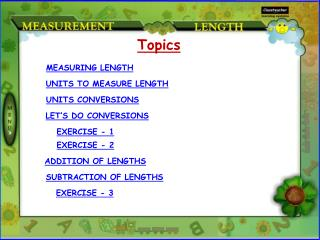DownloadDownload PresentationMEASURING LENGTH

# MEASURING LENGTH

Télécharger la présentation## MEASURING LENGTH

- - - - - - - - - - - - - - - - - - - - - - - - - - - E N D - - - - - - - - - - - - - - - - - - - - - - - - - - -
##### Presentation Transcript

1. Topics MEASURING LENGTH UNITS TO MEASURE LENGTH UNITS CONVERSIONS LET’S DO CONVERSIONS EXERCISE - 1 EXERCISE - 2 ADDITION OF LENGTHS SUBTRACTION OF LENGTHS EXERCISE - 3

2. MEASURING LENGTH Length is a measure of how long or wide something is. Rulers and tape measures can be used to measure length. Rulers Tape

3. UNITS TO MEASURE LENGTH Length is measured in millimeters (mm), centimeters (cm), meters (m) or kilometers (km). Millimeters (mm) and centimeters (cm) are used to measure smaller objects such as: Kilometers is used to measure even longer length such as: Meters is used to measure length of objects such as: Distances traveled by bike Distances between two bus stops Distances between school and home 8 cm 13 mm 7 metres 7.5 metres 5.5 metres 9 mm

4. UNITS CONVERSIONS Centimeter to Millimeters 1 cm = 10 mm Kilometer to Meters 1 km = 1000 m Meter to Centimeters 1 m = 100 cm Kilometer to Centimeters 1 km = 1000 m = 100,000 cm Meter to Millimeters 1 m = 100 cm = 1000 mm

5. LET’S DO CONVERSIONS Point to remember: To convert centimetres to metres, divide by 100. In result, the quotient is metres and the remainder is centimetres. Convert 825 centimetres to metres. To convert metres to kilometres, divide by 1000. In result, the quotient is kilimetres and the remainder is metres. metres Convert 5064 metres into kilometres. kilometres centimetres metres  825 centimetres = 8 metres 25 centimetres.  5064 metres = 50 kilometres 64 metres.

6. EXERCISE - 1 Express in metres and centimetres. 245 centimetres 800 centimetres 905 centimetres 1,008 centimetres 6,542 centimetres 10 metres 8 centimetres 65 metres 42 centimetres 2 metres 45 centimetres 9 metres 5 centimetres 8 metres

7. EXERCISE - 2 Express in kilometres and metres. 25,105 metres 4,505 metres 50,025 metres 55,333 metres 5,005 metres 6,000 metres 25 kilometres 105 metres 50 kilometres 25 metres 4 kilometres 505 metres 5 kilometres 5 metres 6 kilometres 55 kilometres 333 metres

8. ADDITION OF LENGTHS Point to remember: Write kilometres under kilometres, metres under metres, centimetres under centimetres and milimetres under milimetres. Perform simple addition. Example: Add 5 m 75 cm and 12 m 34 cm.

9. SUBTRACTION OF LENGTHS Point to remember: Write kilometres under kilometres, metres under metres, centimetres under centimetres and milimetres under milimetres. Perform simple subtraction. Example: Subtract 5 m 75 cm from 12 m 34 cm.

10. EXERCISE - 3 Add 6 m and 5 m 5 cm. Subtract 53 km 42 m from 75 km 30 m. Add 12 cm 15 mm and 70 cm 5 mm. Add 25 km 40 m and 55 km 78 m. Subtract 5 m 40 cm from 12 m 18 cm. Subtract 22 cm 12 mm from 30 cm. 11 metres 5 cm 82 cm 20 mm 81 km 18 m 6 m 78 cm 7 cm 88 mm 21 km 88 m